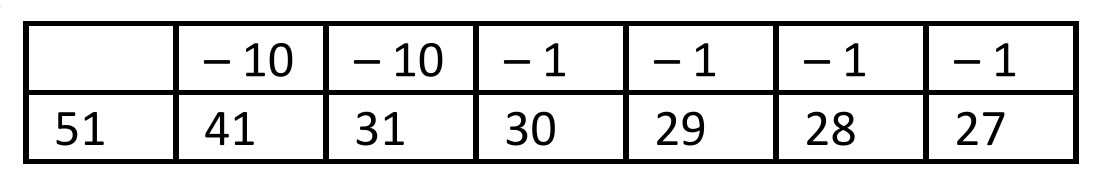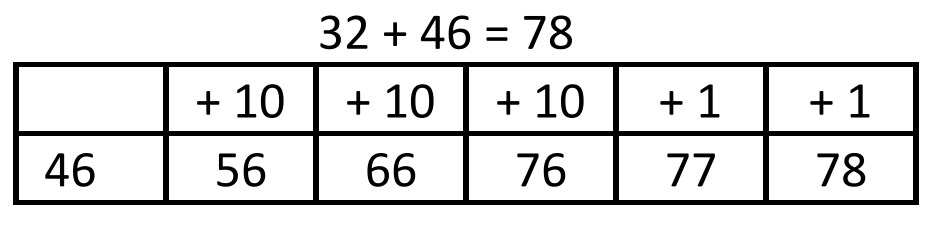# 2nd Grade, Activity 184: Use Place Value Understanding and Properties of Operations to Add and Subtract.

Fluently add and subtract within 100 using strategies based on place value, properties of operations, and/or the relationship between addition and subtraction.

• Group: Pairs
• Materials: paper and pencil, Adding and Subtracting Mentally sheet

Issue a small index card to each student. Ask students to write the following expressions on their cards, one expression per card: + 1, – 1, + 10, – 10. The teacher writes the problem “51 – 24” on the board. Instruct students to write the problem on their papers. To show that 24 is being subtracted from 51, invite two students with minus ten cards (–10) and 4 students with minus one cards (– 1) to write their numbers on the board. Explain that they will use a mental strategy to subtract 24 from 51. Point out that together the numbers on students’ cards are equal to 24. Each student will perform the operation on his or her card to find the difference of the two numbers. Students write the sequence on the board as follows and the class writes the sequence on their papers. Begin by writing 51, have a student subtract 10 twice to take 20 away. Then take 1 away four times to show 4 being subtracted from each number. 51 – 24 = 27Model how to add (32 + 46), start the table with 46, the largest number. Invite 3 students with + 10 cards and 2 students with + 1 cards. (To represent 32) Have students count aloud 10, 20 30, 31, 32,” to show the quantity that is being added to 46 is 32.Model the addition and subtraction problems below in the same manner.

64 – 25

37 + 41

84 + 16

72 – 45